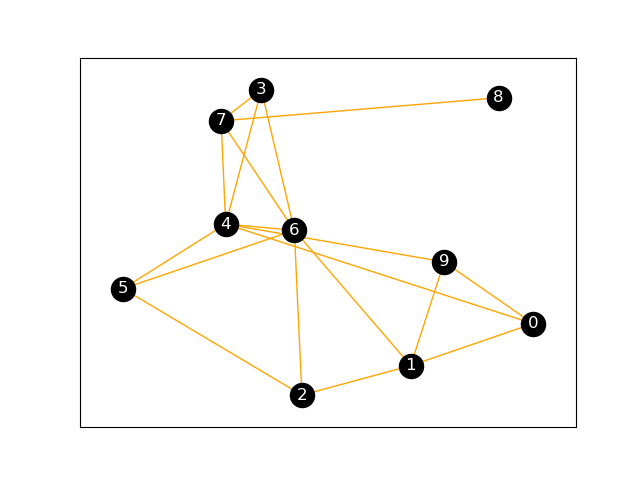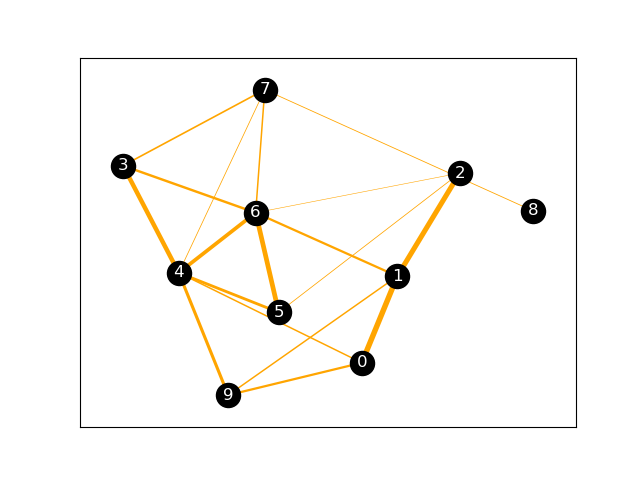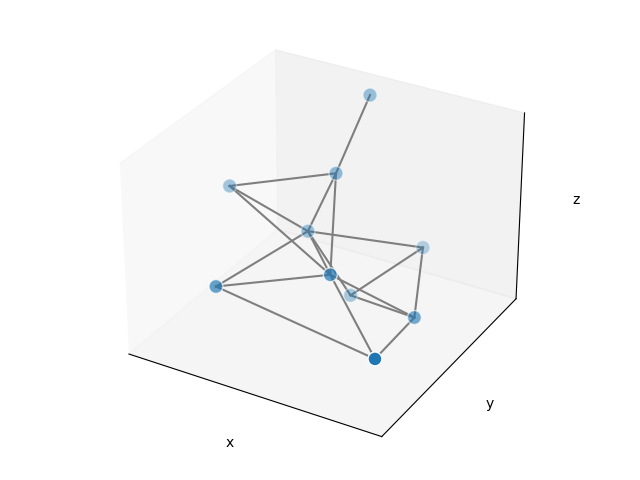# 用NetworkX生成并绘制（带权）无向图

2022-05-11 09:36 176 查看

NetworkX是一个非常强大的网络科学工具，它封装了图的数据结构和许多经典图算法，也内置了许多可视化函数可供调用。

## 1. 随机图生成

`G = nx.erdos_renyi_graph(10, 0.3, seed=1)`

```G = nx.Graph()
for u, v in nx.erdos_renyi_graph(10, 0.3, seed=1).edges():

## 2. 2D布局可视化

`spring_layout`

`pos = nx.spring_layout(G, iterations=20) #我们设算法迭代次数为20次`

```nx.draw_networkx_edges(G, pos, edge_color="orange")
nx.draw_networkx_nodes(G, pos, node_color="black")
nx.draw_networkx_labels(G, pos, font_color="white")
plt.show()``````nx.draw_networkx_edges(G,pos, width=[float(d['weight']*10) for (u,v,d) in G.edges(data=True)], edge_color="orange")
nx.draw_networkx_nodes(G,pos, node_color="black")
nx.draw_networkx_labels(G, pos, font_color="white")
plt.show()```## 3. 3D布局可视化

```# 3d spring layout
pos = nx.spring_layout(G, dim=3, seed=779)
# Extract node and edge positions from the layout
node_xyz = np.array([pos[v] for v in sorted(G)])
edge_xyz = np.array([(pos[u], pos[v]) for u, v in G.edges()])

# Create the 3D figure
fig = plt.figure()

# Plot the nodes - alpha is scaled by "depth" automatically
ax.scatter(*node_xyz.T, s=100, ec="w")

# Plot the edges
for vizedge in edge_xyz:
ax.plot(*vizedge.T, color="tab:gray")

def _format_axes(ax):
"""Visualization options for the 3D axes."""
# Turn gridlines off
ax.grid(False)
# Suppress tick labels
for dim in (ax.xaxis, ax.yaxis, ax.zaxis):
dim.set_ticks([])
# Set axes labels
ax.set_xlabel("x")
ax.set_ylabel("y")
ax.set_zlabel("z")

_format_axes(ax)
fig.tight_layout()
plt.show()```